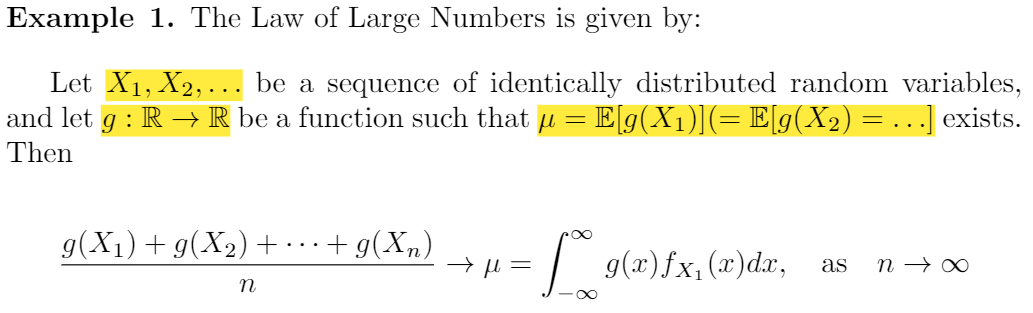# LaTeX: Mathematical Typesetting

This topic guide was created to complement the Introduction to LaTeX workshop conducted by the Library and point users to resources and tips related to using LaTeX and Overleaf.

## Inline Mode

This method is used when you need to add in mathematical symbols as part of a paragraph. Here is an example, highlighted in yellow:Inline mode is simple, all you have to do is bracket your symbols using '$'. E.g. Consider the equation $x+y=z\$ here we can use substitution to...

## Math Mode

Math mode is used when you need equations or formulas to be isolated and not part of any text. Here is an example highlighted in purple:

Desired result Command(s) Notes
x+y=z                                 (1)

\begin{equation}

x+y=z

\end{equation}

This is the simplest version of writing equations in math mode. By default, it will generate an equation number on the right for easy identification.
x+y=z

\begin{equation*}

x+y=z

\end{equation*}

If you do not want or need the equation number at the side, use this instead.

x+y=z                                  (1)

m+n>y                                  (2)

\begin{align}

x+y&=z \\

m+n&>y

\end{align}

If you want to write a system of equations and you want them aligned according to a particular operator (in this case the equals sign), use the align environment and indicate the aligning operator by using ampersand (&).

Note: To remove the equation numbers, use align* instead.

Note 2: If you do not care about alignment, use gather environment instead.